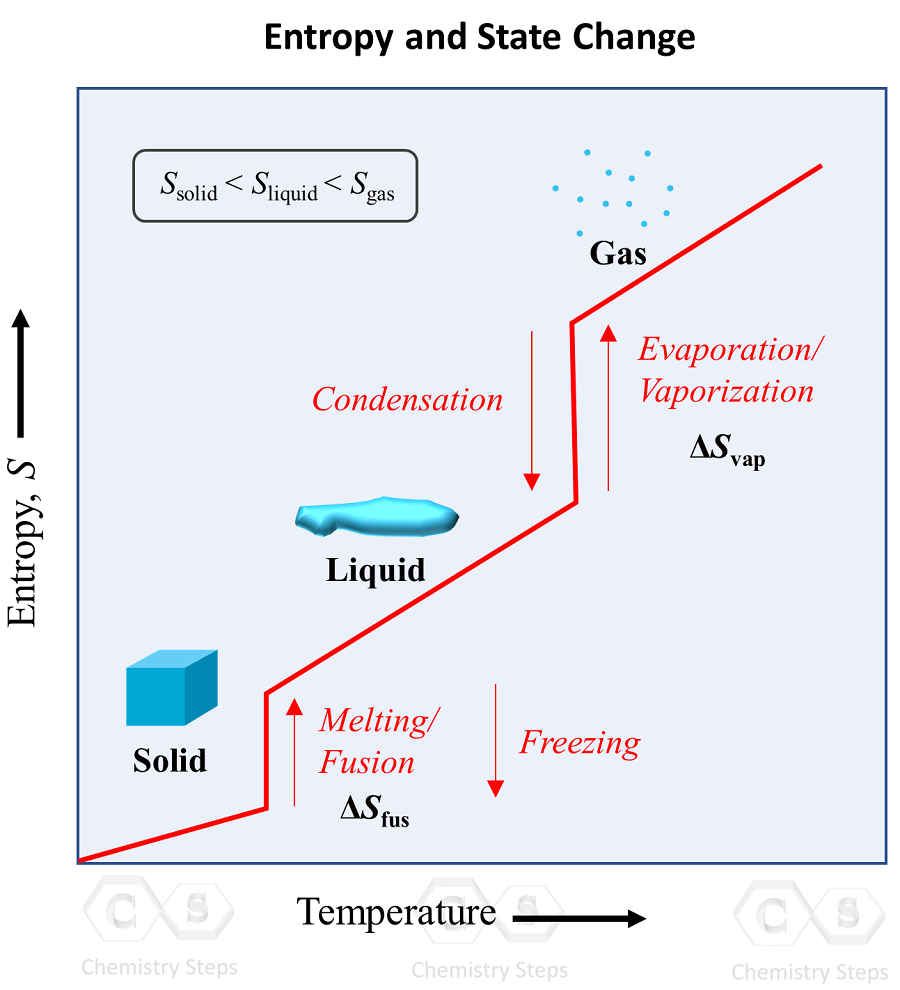## Chemical Thermodynamics

On the basis of determining the entropy change associated with phase transitions is the third law of thermodynamics: the entropy of a perfect crystalline substance is zero at the absolute zero temperature.

An implication of this is that the entropy is the lowest in solids, and it keeps increasing in the order of going to liquid and gas states: solid < liquid < gas. This behavior is explained by the increasing freedom of motion when molecules go from the most ordered solid state to liquid, and then a gas state where the degree of randomness is the highest.

The phase transition graph showing the entropy vs temperature is very useful to visualize this concept:Notice how the entropy is still increasing in the regions where the temperature is not changing. These are the points of phase transitions where, for example, the liquid turns into a gas even at the same temperature. Remember this pattern, and the corresponding terms for each pair of opposite processes: melting/fusion vs freezing, evaporation/vaporization vs condensation.

# Calculating the Entropy Change of State Changes

To derive an equation for the entropy change associated with phase transitions, we need to remember that these changes occur when the system is at equilibrium. Remember, also that when the system is at equilibrium the Gibbs free energy is equal to zero:

ΔG = ΔH – TΔS = 0

Rearranging the equation, we get an expression for ΔS:

$\Delta S\, = \,\frac{{\Delta H}}{T}$

ΔS is the entropy change of the system measured in J/K, and T – the temperature in Kelvin

The entropy change can be calculated using this equation as long as the temperature remains constant.

For example, Chloroform, CHCl3, is a common organic solvent once used as an anesthetic. a) Given that the heat of vaporization of chloroform is 29.24 kJ/mol, calculate the entropy change, S of the system when 1.00 mol of CHCl3 evaporates at its boiling point (61.2 °C). b) What is the entropy of the gaseous chloroform at this temperature if the standard entropy of chloroform is 295.6 J/(mol K)?

Solution:

$\Delta S\; = \,\frac{{\Delta {H_{vap}}}}{T}\, = \;\frac{{{\rm{29}}{\rm{.24 kJ/mol}}}}{{(61.2\, + \,273)\,K}}\; = \,0.0875\,{\rm{kJ/K }} \cdot \,{\rm{mol}}\,{\rm{ = }}\,{\rm{87}}{\rm{.5}}\;{\rm{J/K }} \cdot \,{\rm{mol}}\,$

To find the entropy of chloroform in the gas state, we need to add the ΔS to its entropy at the standard, liquid state:

S (g) = S (l) + ΔS = 295.6 J/(mol K) + 87.5 J/(mol K) = 383.1 J/(mol K)

# Reversible Processes

Because at constant pressure, the enthalpy change is equal to the heat exchange between the system and the surroundings, we can write the equation for ΔS as:

$\Delta S\, = \,\frac{{{q_{{\rm{rev}}}}}}{T}$

qrev is the heat exchanged with the surroundings in a reversible process

A reversible process is one that reverses direction upon an infinitesimally small change in some property. For example, the melting of ice at 0 oC is a reversible process because an infinitesimally small removal of heat reverses the melting and causes freezing instead. On the other hand, the melting of ice at room temperature is irreversible because removing a very tiny amount of heat does not reverse the melting.

With the same principle, the vaporization/condensation process is also reversible. Therefore, this is a good explanation as to why the enthalpy changes associated with these transitions are related with a reverse sign: ΔHcond = – ΔHvap, ΔHmelt = – ΔHfreez.

For example, Liquid nitrogen has many applications in chemical laboratories. Calculate the change in entropy, ΔS that occurs in the system when 8.60 mole of nitrogen condenses from a gas to a liquid at its boiling point (-196 °C). The heat of the vaporization of nitrogen is 2.7928 kJ/mol.

Solution: Let’s first calculate the heat of vaporization of 8.60 mol nitrogen:

$q\;{\rm{ = }}\,{\rm{8}}{\rm{.60}}\,\cancel{{{\rm{mol}}}}\,{\rm{ \times }}\,\frac{{2.7928\,{\rm{kJ}}}}{{\cancel{{{\rm{mol}}}}}}{\rm{ = }}\,{\rm{24}}{\rm{.0 kJ}}$

Notice here that we have a condensation which is the opposite process of vaporization, therefore, we need to change the sign of ΔH as ΔHcond = −ΔHvap:

$\Delta S\; = \,\frac{{ – \Delta {H_{vap}}}}{T}\, = \;\frac{{{\rm{ – 24}}{\rm{.0 kJ/mol}}}}{{( – 196\, + \,273)\,K}}\; = \, – 0.312\,{\rm{kJ/K }} \cdot \,{\rm{mol}}\,{\rm{ = }}\, – {\rm{312}}\;{\rm{J/K }} \cdot \,{\rm{mol}}\,$

The entropy change is negative which is what we expect when going from a gas to a liquid state.

Check Also

#### Practice

1.

Determine the change in entropy, ΔS that occurs in the system when 1.00 mole of benzene (C6H6) melts at its melting point (5.5 °C). The heat of fusion for benzene is 9.92 kJ/mol.

Solution
This content is available to registered users only. Click here to Register! By joining Chemistry Steps, you will gain instant access to the answers and solutions for all the Practice Problems and the powerful set of General Chemistry 1 and 2 Summary Study Guides.
2.

Isopropanol (C3H8O) is used as rubbing alcohol. The heat of vaporization of isopropanol at its boiling point (82.5 oC) is 39.9 kJ/mol. What is the entropy change, ΔS when 1.30 mol C3H8O vaporizes at its boiling point?